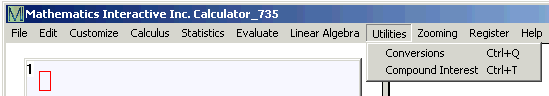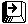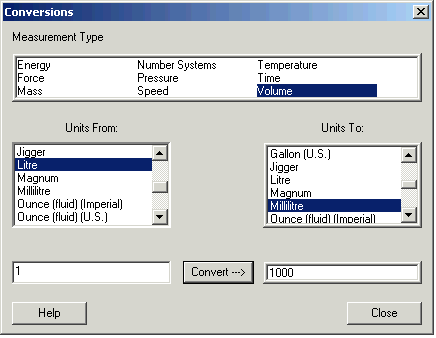# Volume Conversions

A wide variety of units are used to measure volume.

You can convert volume measured in one unit to another unit by clicking Utilities and then Conversions.Holding the Control key and typing q has the same result.

In the Conversions window, select Volume. If you can't see the word Volume in the Measurement Type box, hit thearrow key a few times.Click the unit you have in the Units From: box, and the units you want in the Units To: box.

Enter the number in the lower left box and click Convert.

This window shows that 1 litre is 1000 millilitres.

The conversions are based on:

1 cubic meter = 1 000 000 cubic centimeters

1 cubic centimeter = 1 milliliter

1 cubic foot = 1728 cubic inches = 0.028 316 846 592 cubic meter

1 cubic yard = 27 cubic feet

1 barrel = 42 gallons (US)

1 gallon (US) = 4 quarts (US) = 8 pints (US) = 16 cups (US)

1 gallon (Imperial) = 4 quarts (Imperial) = 8 pints (Imperial) = 16 cups (Imperial)

1 jigger = 1.5 fluid ounces (US)

1 tablespoon (US) = 3 teaspoons (US)

1 tablespoon (Imperial) = 3 teaspoons (US)

1 fluid ounce (US) = 6 teaspoons (US) = 29.573 529 5625 millilitres

1 fluid ounce (Imperial) = 4.8 teaspoons (Imperial) = 28.413 0625 millilitres

1 magnum = 1.5 litres